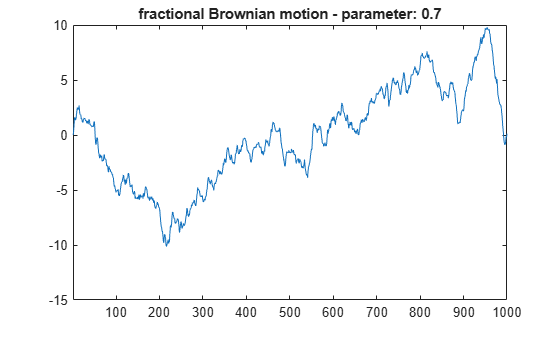# wfbm

Fractional Brownian motion synthesis

## Syntax

``fBm = wfbm(H,L)``
``fBm = wfbm(H,L,ns,w)``
``fBm = wfbm(H,L,'plot')``

## Description

example

````fBm = wfbm(H,L)` returns a fractional Brownian motion signal `fBm` of the Hurst parameter `H` (```0 < H < 1```) and length `L`, following the algorithm proposed by Abry and Sellan . By default, `wfbm` uses six reconstruction steps and the orthogonal `db10` wavelet.```
````fBm = wfbm(H,L,ns,w)` returns the signal using `ns` reconstruction steps and the sufficiently regular orthogonal wavelet `w`.`fBm = wfbm(H,L,w,ns)` is equivalent to ```fBm = wfbm(H,L,ns,w)```.```
````fBm = wfbm(H,L,'plot')` generates and plots the `fBm` signal. The following syntaxes also generate and plot the signal. `fBm = wfbm(H,L,'plot',w)``fBm = wfbm(H,L,'plot',ns)``fBm = wfbm(H,L,'plot',w,ns)``fBm = wfbm(H,L,'plot',ns,w)` ```

## Examples

collapse all

According to the value of the Hurst parameter `H`, the `fBm` exhibits for `H > 0.5`, long-range dependence and for `H < 0.5`, short or intermediate dependence. This example shows each situation using the `wfbm` function, which generates a sample path of this process.

For purposes of reproducibility, set the random seed to the default value. Generate a fractional Brownian motion signal of length 1000 with the Hurst parameter of 0.3. Plot the signal.

```rng default h = 0.3; l = 1000; fBm03 = wfbm(h,l,'plot');```Now generate a fractional Brownian motion signal of length 1000 with the Hurst parameter of 0.7. The signal clearly exhibits a stronger low-frequency component and has, locally, less irregular behavior than `fBm03`.

```h = 0.7; l = 1000; fBm07 = wfbm(h,l,'plot');```## Input Arguments

collapse all

Hurst parameter, specified as a positive scalar strictly less than 1.

Example: `fBm = wfbm(0.4,1000)` generates a fractional Brownian motion of length `L = 1000` with Hurst parameter `H = 0.4`.

Data Types: `double`

Signal length, specified as a positive integer strictly greater than 100.

Example: `fBm = wfbm(0.1,500)` generates a fractional Brownian motion of length `L = 500` with Hurst parameter `H = 0.1`.

Data Types: `double`

Number of reconstruction steps, specified as a positive integer greater than 1.

Data Types: `double`

Orthogonal wavelet recognized by `wavemngr`, specified as a character vector or string scalar.

## Output Arguments

collapse all

Fractional Brownian motion signal, returned as a vector of length `L`.

collapse all

### Fractional Brownian Motion

A fractional Brownian motion (`fBm`) is a continuous-time Gaussian process depending on the Hurst parameter ```0 < H < 1```. It generalizes the ordinary Brownian motion corresponding to `H = 0.5` and whose derivative is the white noise. The `fBm` is self-similar in distribution and the variance of the increments is given by

```Var(fBm(t)-fBm(s)) = v |t-s|^(2H) ```

where `v` is a positive constant.

## Algorithms

Starting from the expression of the `fBm` process as a fractional integral of the white noise process, the idea of the algorithm is to build a biorthogonal wavelet depending on a given orthogonal one and adapted to the parameter `H`.

Then the generated sample path is obtained by the reconstruction using the new wavelet starting from a wavelet decomposition at a given level designed as follows: details coefficients are independent random Gaussian realizations and approximation coefficients come from a fractional ARIMA process.

This method was first proposed by Meyer and Sellan and implementation issues were examined by Abry and Sellan .

Nevertheless, the samples generated following this original scheme exhibit too many high-frequency components. To circumvent this undesirable behavior Bardet et al.  propose downsampling the obtained sample by a factor of 10.

Two internal parameters `delta = 10` (the downsampling factor) and a threshold `prec = 1E-4`, to evaluate series by truncated sums, can be modified by the user for extreme values of `H`.

A complete overview of long-range dependence process generators is available in Bardet et al .

 Abry, Patrice, and Fabrice Sellan. “The Wavelet-Based Synthesis for Fractional Brownian Motion Proposed by F. Sellan and Y. Meyer: Remarks and Fast Implementation.” Applied and Computational Harmonic Analysis 3, no. 4 (October 1996): 377–83. https://doi.org/10.1006/acha.1996.0030.

 Bardet, Jean-Marc, Gabriel Lang, Georges Oppenheim, Anne Philippe, Stilian Stoev, and Murad S. Taqqu. “Generators of Long-Range Dependent Processes: A Survey.” In Theory and Applications of Long-Range Dependence, edited by Paul Doukhan, Georges Oppenheim, and Murad S. Taqqu, 579–623. Boston: Birkhauser, 2003.